Anzeige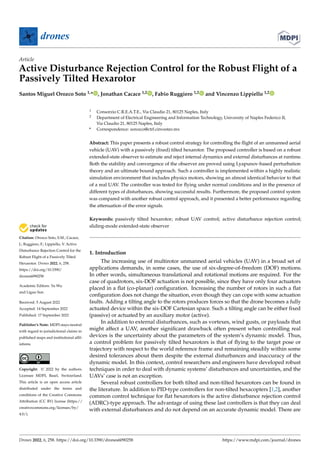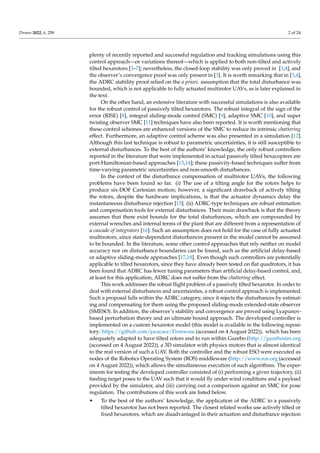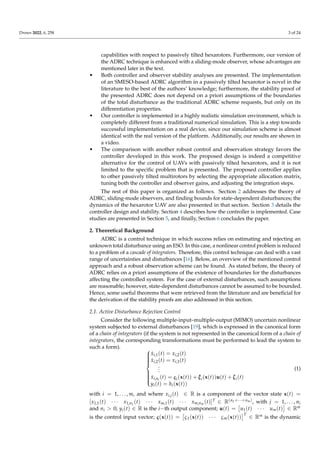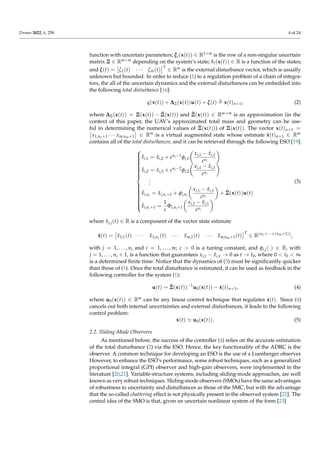Anzeige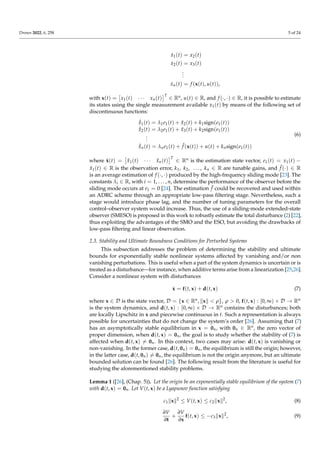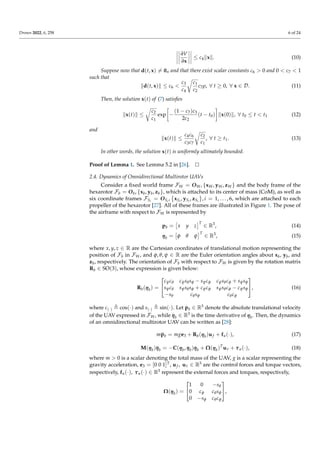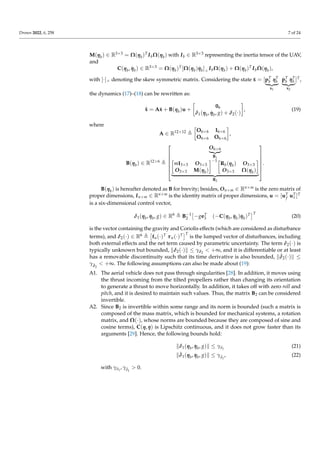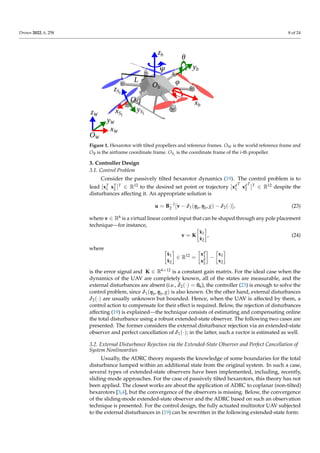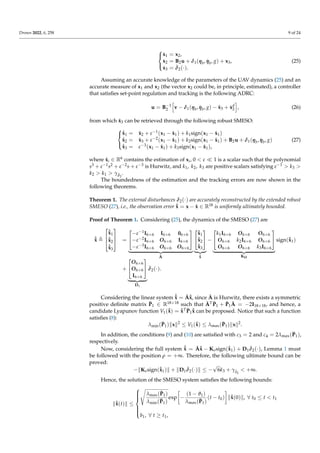Anzeige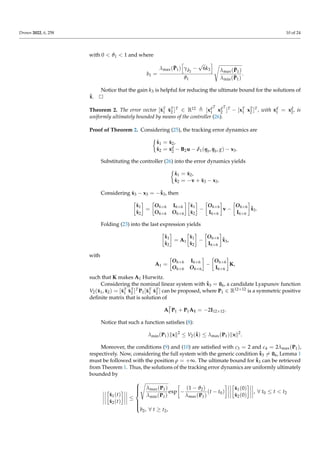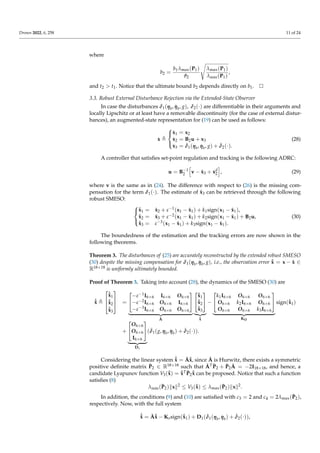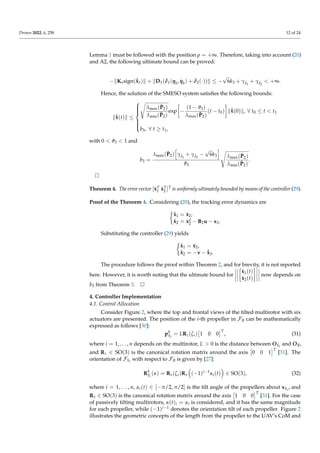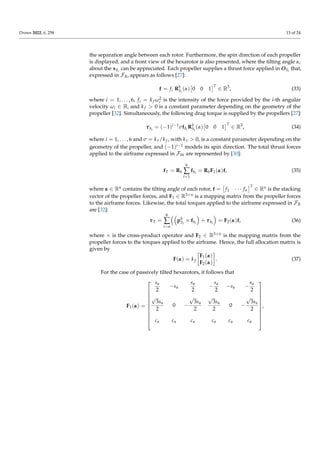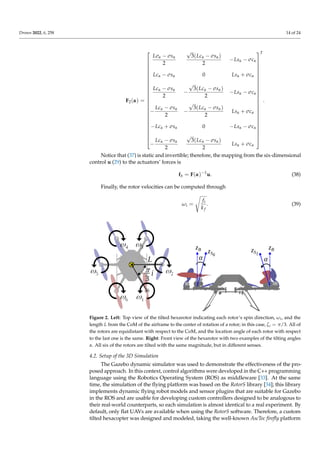Anzeige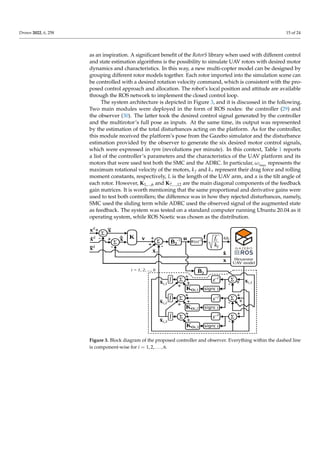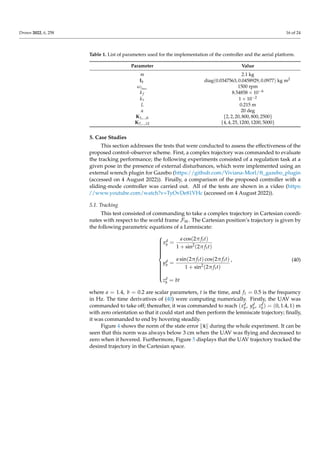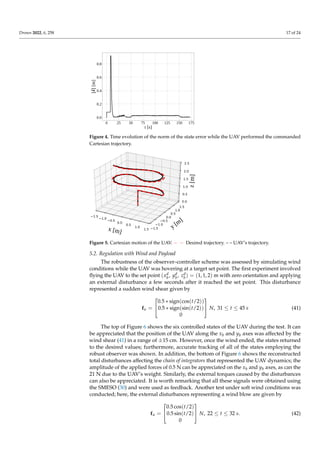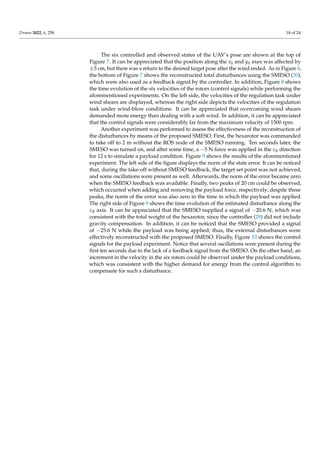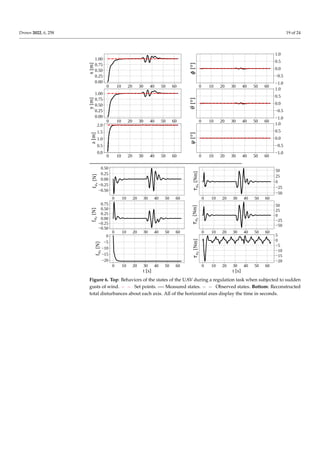Anzeige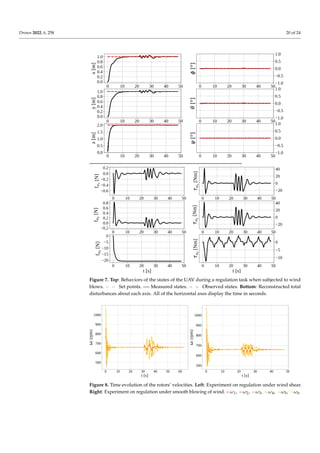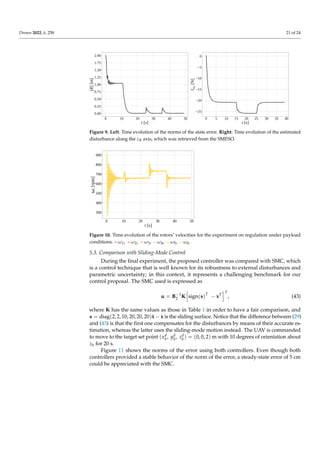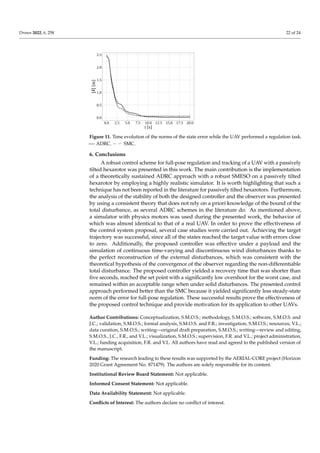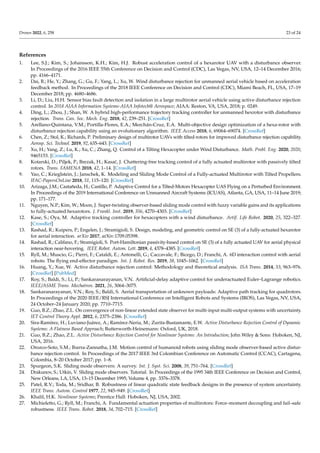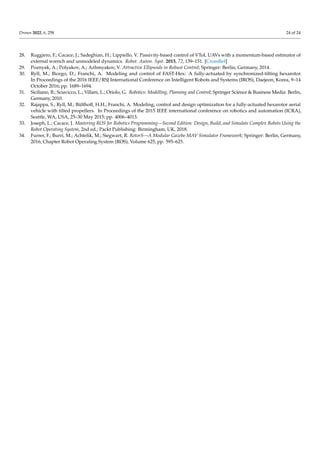Nächste SlideShare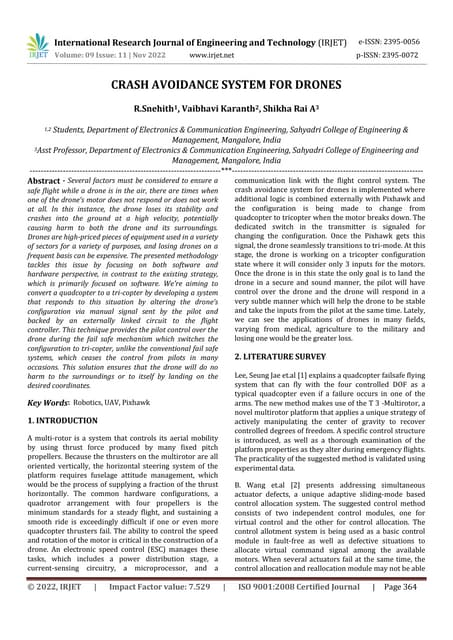CRASH AVOIDANCE SYSTEM FOR DRONES
1 von 24
Anzeige

### drones-06-00258-v2.pdf

4. Drones 2022, 6, 258 4 of 24 function with uncertain parameters; ξi(x(t)) ∈ R1×m is the row of a non-singular uncertain matrix Ξ ∈ Rm×m depending on the system’s state; hi(x(t)) ∈ R is a function of the states; and ζ(t) = ζ1(t) · · · ζm(t) T ∈ Rm is the external disturbance vector, which is usually unknown but bounded. In order to reduce (1) to a regulation problem of a chain of integra- tors, the all of the uncertain dynamics and the external disturbances can be embedded into the following total disturbance : ς(x(t)) + ∆Ξ(x(t))u(t) + ζ(t) , x(t)n+1, (2) where ∆Ξ(x(t)) = Ξ(x(t)) − e Ξ(x(t)) and e Ξ(x(t)) ∈ Rm×m is an approximation (in the context of this paper, the UAV’s approximated total mass and geometry can be use- ful in determining the numerical values of e Ξ(x(t))) of Ξ(x(t)). The vector x(t)n+1 = x1,n1+1 · · · xm,nm+1 ∈ Rm is a virtual augmented state whose estimate x̂(t)n+1 ∈ Rm contains all of the total disturbances, and it can be retrieved through the following ESO :                              ˙ x̂i,1 = x̂i,2 + εni−1φi,1 xi,1 − x̂i,1 εni ˙ x̂i,2 = x̂i,3 + εni−2φi,2 xi,1 − x̂i,1 εni . . . ˙ x̂i,ni = x̂i,ni+1 + φi,ni xi,1 − x̂i,1 εni + e Ξ(x(t))u(t) ˙ x̂i,ni+1 = 1 ε Φi,ni+1 xi,1 − x̂i,1 εni (3) where x̂i,j(t) ∈ R is a component of the vector state estimate x̂(t) = x̂1,1(t) · · · x̂1,n1 (t) · · · x̂m,1(t) · · · x̂m,nm+1(t) T ∈ R(n1+···+(nm+1)) , with j = 1, . . . , ni and i = 1, . . . , m; ε 0 is a tuning constant, and φi,j(·) ∈ R, with j = 1, . . . , ni + 1, is a function that guarantees xi,1 − x̂i,1 → 0 as t → t0, where 0 t0 ∞ is a determined finite time. Notice that the dynamics of (3) must be significantly quicker than those of (1). Once the total disturbance is estimated, it can be used as feedback in the following controller for the system (1): u(t) = e Ξ(x(t))−1 u0(x(t)) − x̂(t)n+1, (4) where u0(x(t)) ∈ Rm can be any linear control technique that regulates ẋ(t). Since (4) cancels out both internal uncertainties and external disturbances, it leads to the following control problem: ẋ(t) ' u0(x(t)). (5) 2.2. Sliding-Mode Observers As mentioned before, the success of the controller (4) relies on the accurate estimation of the total disturbance (2) via the ESO. Hence, the key functionality of the ADRC is the observer. A common technique for developing an ESO is the use of a Luenberger observer. However, to enhance the ESO’s performance, some robust techniques, such as a generalized proportional integral (GPI) observer and high-gain observers, were implemented in the literature [20,21]. Variable-structure systems, including sliding-mode approaches, are well known as very robust techniques. Sliding-mode observers (SMOs) have the same advantages of robustness to uncertainty and disturbances as those of the SMC, but with the advantage that the so-called chattering effect is not physically present in the observed system . The central idea of the SMO is that, given an uncertain nonlinear system of the form 
5. Drones 2022, 6, 258 5 of 24 ẋ1(t) = x2(t) ẋ2(t) = x3(t) . . . ẋn(t) = f (x(t), u(t)), with x(t) = x1(t) · · · xn(t) T ∈ Rn, u(t) ∈ R, and f (·, ·) ∈ R, it is possible to estimate its states using the single measurement available x1(t) by means of the following set of discontinuous functions: ˙ x̂1(t) = λ1e1(t) + x̂2(t) + k1sign(e1(t)) ˙ x̂2(t) = λ2e1(t) + x̂3(t) + k2sign(e1(t)) . . . ˙ x̂n(t) = λne1(t) + ˆ f (x(t)) + u(t) + knsign(e1(t)) (6) where x̂(t) = x̂1(t) · · · x̂n(t) T ∈ Rn is the estimation state vector, e1(t) = x1(t) − x̂1(t) ∈ R is the observation error, k1, k2, . . . , kn ∈ R are tunable gains, and ˆ f (·) ∈ R is an average estimation of f (·, ·) produced by the high-frequency sliding mode . The constants λi ∈ R, with i = 1, . . . , n, determine the performance of the observer before the sliding mode occurs at e1 = 0 . The estimation ˆ f could be recovered and used within an ADRC scheme through an appropriate low-pass filtering stage. Nevertheless, such a stage would introduce phase lag, and the number of tuning parameters for the overall control–observer system would increase. Thus, the use of a sliding-mode extended-state observer (SMESO) is proposed in this work to robustly estimate the total disturbance (2) , thus exploiting the advantages of the SMO and the ESO, but avoiding the drawbacks of low-pass filtering and linear observation. 2.3. Stability and Ultimate Boundness Conditions for Perturbed Systems This subsection addresses the problem of determining the stability and ultimate bounds for exponentially stable nonlinear systems affected by vanishing and/or non vanishing perturbations. This is useful when a part of the system dynamics is uncertain or is treated as a disturbance—for instance, when additive terms arise from a linearization [25,26]. Consider a nonlinear system with disturbances ẋ = f(t, x) + d(t, x) (7) where x ∈ D is the state vector, D = {x ∈ Rn, kxk ρ}, ρ 0, f(t, x) : [0, ∞) × D → Rn is the system dynamics, and d(t, x) : [0, ∞) × D → Rn contains the disturbances; both are locally Lipschitz in x and piecewise continuous in t. Such a representation is always possible for uncertainties that do not change the system’s order . Assuming that (7) has an asymptotically stable equilibrium in x = 0n, with 0n ∈ Rn, the zero vector of proper dimension, when d(t, x) = 0n, the goal is to study whether the stability of (7) is affected when d(t, x) 6= 0n. In this context, two cases may arise: d(t, x) is vanishing or non-vanishing. In the former case, d(t, 0n) = 0n, the equilibrium is still the origin; however, in the latter case, d(t, 0n) 6= 0n, the equilibrium is not the origin anymore, but an ultimate bounded solution can be found . The following result from the literature is useful for studying the aforementioned stability problems. Lemma 1 (, (Chap. 5)). Let the origin be an exponentially stable equilibrium of the system (7) with d(t, x) = 0n. Let V(t, x) be a Lyapunov function satisfying c1kxk2 ≤ V(t, x) ≤ c2kxk2 , (8) ∂V ∂t + ∂V ∂x f(t, x) ≤ −c3kxk2 , (9)
6. Drones 2022, 6, 258 6 of 24 ∂V ∂x ≤ c4kxk. (10) Suppose now that d(t, x) 6= 0n and that there exist scalar constants c6 0 and 0 c7 1 such that kd(t, x)k ≤ c6 c3 c4 r c1 c2 c7ρ, ∀ t ≥ 0, ∀ x ∈ D. (11) Then, the solution x(t) of (7) satisfies kx(t)k ≤ r c2 c1 exp − (1 − c7)c3 2c2 (t − t0) kx(0)k, ∀ t0 ≤ t t1 (12) and kx(t)k ≤ c4c6 c3c7 r c2 c1 , ∀ t ≥ t1. (13) In other words, the solution x(t) is uniformly ultimately bounded. Proof of Lemma 1. See Lemma 5.2 in . 2.4. Dynamics of Omnidirectional Multirotor UAVs Consider a fixed world frame FW = OW, {xW, yW, zW} and the body frame of the hexarotor Fb = Ob, {xb, yb, zb}, which is attached to its center of mass (CoM), as well as six coordinate frames FSi = OSi , xSi , ySi , zSi , i = 1, . . . , 6, which are attached to each propeller of the hexarotor . All of these frames are illustrated in Figure 1. The pose of the airframe with respect to FW is represented by pb = x y z T ∈ R3 , (14) ηb = φ θ ψ T ∈ R3 , (15) where x, y, z ∈ R are the Cartesian coordinates of translational motion representing the position of Fb in FW, and φ, θ, ψ ∈ R are the Euler orientation angles about xb, yb, and zb, respectively. The orientation of Fb with respect to FW is given by the rotation matrix Rb ∈ SO(3), whose expression is given below: Rb(ηb) =   cψcθ cψsθsφ − sψcφ cψsθcφ + sψsφ sψcθ sψsθsφ + cψcφ sψsθcφ − cψsφ −sθ cθsφ cθcφ  , (16) where c(·) , cos(·) and s(·) , sin(·). Let ṗb ∈ R3 denote the absolute translational velocity of the UAV expressed in FW, while η̇b ∈ R3 is the time derivative of ηb. Then, the dynamics of an omnidirectional multirotor UAV can be written as : mp̈b = mge3 + Rb(ηb)uf + fu(·), (17) M(ηb)η̈b = −C(ηb, η̇b)η̇b + Ω(ηb)T uτ + τu(·), (18) where m 0 is a scalar denoting the total mass of the UAV, g is a scalar representing the gravity acceleration, e3 = [0 0 1]T, uf , uτ ∈ R3 are the control forces and torque vectors, respectively, fu(·), τu(·) ∈ R3 represent the external forces and torques, respectively, Ω(ηb) =   1 0 −sθ 0 cφ cθsφ 0 −sφ cθcφ  ,
7. Drones 2022, 6, 258 7 of 24 M(ηb) ∈ R3×3 = Ω(ηb)T IbΩ(ηb) with Ib ∈ R3×3 representing the inertia tensor of the UAV, and C(ηb, η̇b) ∈ R3×3 = Ω(ηb)T[Ω(ηb)η̇b]× IbΩ(ηb) + Ω(ηb)T IbΩ̇(ηb), with [·]× denoting the skew symmetric matrix. Considering the state x̄ = [pT b ηT b | {z } x1 ṗT b η̇T b | {z } x2 ]T, the dynamics (17)–(18) can be rewritten as: ˙ x̄ = Ax̄ + B(ηb)u + 06 δ1(ηb, η̇b, g) + δ2(·) , (19) where A ∈ R12×12 , O6×6 I6×6 O6×6 O6×6 , B(ηb) ∈ R12×6 ,        O6×6 | {z } B1 mI3×3 O3×3 O3×3 M(ηb) −1 Rb(ηb) O3×3 O3×3 Ω(ηb) | {z } B2        . B(ηb) is hereafter denoted as B for brevity; besides, On×m ∈ Rn×m is the zero matrix of proper dimensions, In×m ∈ Rn×m is the identity matrix of proper dimensions, u = [uT f uT τ ]T is a six-dimensional control vector, δ1(ηb, η̇b, g) ∈ R6 , B−1 2 −geT 3 (−C(ηb, η̇b)η̇b)T T (20) is the vector containing the gravity and Coriolis effects (which are considered as disturbance terms), and δ2(·) ∈ R6 , fu(·)T τu(·)T T is the lumped vector of disturbances, including both external effects and the net term caused by parametric uncertainty. The term δ2(·) is typically unknown but bounded, kδ2(·)k ≤ γδ2 +∞, and it is differentiable or at least has a removable discontinuity such that its time derivative is also bounded, kδ̇2(·)k ≤ γδ̇2 +∞. The following assumptions can also be made about (19): A1. The aerial vehicle does not pass through singularities . In addition, it moves using the thrust incoming from the tilted propellers rather than changing its orientation to generate a thrust to move horizontally. In addition, it takes off with zero roll and pitch, and it is desired to maintain such values. Thus, the matrix B2 can be considered invertible. A2. Since B2 is invertible within some range and its norm is bounded (such a matrix is composed of the mass matrix, which is bounded for mechanical systems, a rotation matrix, and Ω(·), whose norms are bounded because they are composed of sine and cosine terms), C(η, η̇) is Lipschitz continuous, and it does not grow faster than its arguments . Hence, the following bounds hold: kδ1(ηb, η̇b, g)k ≤ γδ1 (21) kδ̇1(ηb, η̇b, g)k ≤ γδ̇1 , (22) with γδ1 , γδ̇1 0.
8. Drones 2022, 6, 258 8 of 24 Figure 1. Hexarotor with tilted propellers and reference frames. OW is the world reference frame and OB is the airframe coordinate frame. OSi is the coordinate frame of the i-th propeller. 3. Controller Design 3.1. Control Problem Consider the passively tilted hexarotor dynamics (19). The control problem is to lead [xT 1 xT 2 ]T ∈ R12 to the desired set point or trajectory [xd 1 T xd 2 T ]T ∈ R12 despite the disturbances affecting it. An appropriate solution is u = B−1 2 [v − δ1(ηb, η̇b, g) − δ2(·)], (23) where v ∈ R6 is a virtual linear control input that can be shaped through any pole placement technique—for instance, v = K x̃1 x̃2 , (24) where x̃1 x̃2 ∈ R12 = xd 1 xd 2 − x1 x2 is the error signal and K ∈ R6×12 is a constant gain matrix. For the ideal case when the dynamics of the UAV are completely known, all of the states are measurable, and the external disturbances are absent (i.e., δ̇2(·) = 06), the controller (23) is enough to solve the control problem, since δ1(ηb, η̇b, g) is also known. On the other hand, external disturbances δ2(·) are usually unknown but bounded. Hence, when the UAV is affected by them, a control action to compensate for their effect is required. Below, the rejection of disturbances affecting (19) is explained—the technique consists of estimating and compensating online the total disturbance using a robust extended-state observer. The following two cases are presented: The former considers the external disturbance rejection via an extended-state observer and perfect cancellation of δ1(·); in the latter, such a vector is estimated as well. 3.2. External Disturbance Rejection via the Extended-State Observer and Perfect Cancellation of System Nonlinearities Usually, the ADRC theory requests the knowledge of some boundaries for the total disturbance lumped within an additional state from the original system. In such a case, several types of extended-state observers have been implemented, including, recently, sliding-mode approaches. For the case of passively tilted hexarotors, this theory has not been applied. The closest works are about the application of ADRC to coplanar (non-tilted) hexarotors [3,4], but the convergence of the observers is missing. Below, the convergence of the sliding-mode extended-state observer and the ADRC based on such an observation technique is presented. For the control design, the fully actuated multirotor UAV subjected to the external disturbances in (19) can be rewritten in the following extended-state form:
9. Drones 2022, 6, 258 9 of 24    ẋ1 = x2, ẋ2 = B2u + δ1(ηb, η̇b, g) + x3, ẋ3 = δ̇2(·). (25) Assuming an accurate knowledge of the parameters of the UAV dynamics (25) and an accurate measure of x1 and x2 (the vector x2 could be, in principle, estimated), a controller that satisfies set-point regulation and tracking is the following ADRC: u = B−1 2 h v − δ1(ηb, η̇b, g) − x̂3 + ẋd 2 i , (26) from which x̂3 can be retrieved through the following robust SMESO:    ˙ x̂1 = x̂2 + ε−1(x1 − x̂1) + k1sign(x1 − x̂1) ˙ x̂2 = x̂3 + ε−2(x1 − x̂1) + k2sign(x1 − x̂1) + B2u + δ1(ηb, η̇b, g) ˙ x̂3 = ε−3(x1 − x̂1) + k3sign(x1 − x̂1), (27) where x̂i ∈ R6 contains the estimation of xi, 0 ε 1 is a scalar such that the polynomial s3 + ε−1s2 + ε−2s + ε−3 is Hurwitz, and k1, k2, k3 are positive scalars satisfying ε−1 k3 k2 k1 γδ̇2 . The boundedness of the estimation and the tracking errors are now shown in the following theorems. Theorem 1. The external disturbances δ2(·) are accurately reconstructed by the extended robust SMESO (27), i.e., the observation error ˆ x̃ = x − x̂ ∈ R18 is uniformly ultimately bounded. Proof of Theorem 1. Considering (25), the dynamics of the SMESO (27) are ˙ ˆ x̃ ,    ˙ ˆ x̃1 ˙ ˆ x̃2 ˙ ˆ x̃3    =   −ε−1I6×6 I6×6 06×6 −ε−2I6×6 O6×6 I6×6 −ε−3I6×6 O6×6 O6×6   | {z } Ã   ˆ x̃1 ˆ x̃2 ˆ x̃3   | {z } ˆ x̃ −   k1I6×6 O6×6 O6×6 O6×6 k2I6×6 O6×6 O6×6 O6×6 k3I6×6   | {z } KO sign(ˆ x̃1) +   O6×6 O6×6 I6×6   | {z } D1 δ̇2(·). Considering the linear system ˙ ˆ x̃ = Ãˆ x̃, since Ã is Hurwitz, there exists a symmetric positive definite matrix P̃1 ∈ R18×18 such that ÃTP̃1 + P̃1Ã = −2I18×18, and hence, a candidate Lyapunov function V1(ˆ x̃) = ˆ x̃TP̃1 ˆ x̃ can be proposed. Notice that such a function satisfies (8): λmin(P̃1)kxk2 ≤ V1(ˆ x̃) ≤ λmax(P̃1)kxk2 . In addition, the conditions (9) and (10) are satisfied with c3 = 2 and c4 = 2λmax(P̃1), respectively. Now, considering the full system ˙ ˆ x̃ = Ãˆ x̃ − Kosign(ˆ x̃1) + D1δ̇2(·), Lemma 1 must be followed with the position ρ = +∞. Therefore, the following ultimate bound can be proved: −kKosign(ˆ x̃1)k + kD1δ̇2(·)k ≤ − √ 6k3 + γδ̇2 +∞. Hence, the solution of the SMESO system satisfies the following bounds: kˆ x̃(t)k ≤          s λmax(P̃1) λmin(P̃1) exp − (1 − ϑ1) λmax(P̃1) (t − t0) kˆ x̃(0)k, ∀ t0 ≤ t t1 b1, ∀ t ≥ t1,
10. Drones 2022, 6, 258 10 of 24 with 0 ϑ1 1 and where b1 = λmax(P̃1) h γδ̇2 − √ 6k3 i ϑ1 s λmax(P̃1) λmin(P̃1) . Notice that the gain k3 is helpful for reducing the ultimate bound for the solutions of ˆ x̃. Theorem 2. The error vector [x̃T 1 x̃T 2 ]T ∈ R12 , [xd 1 T xd 2 T ]T − [xT 1 xT 2 ]T, with ẋd 1 = xd 2, is uniformly ultimately bounded by means of the controller (26). Proof of Theorem 2. Considering (25), the tracking error dynamics are ˙ x̃1 = x̃2, ˙ x̃2 = ẋd 2 − B2u − δ1(ηb, η̇b, g) − x3. Substituting the controller (26) into the error dynamics yields ˙ x̃1 = x̃2, ˙ x̃2 = −v + x̂3 − x3. Considering x̂3 − x3 = −ˆ x̃3, then ˙ x̃1 ˙ x̃2 = O6×6 I6×6 O6×6 O6×6 x̃1 x̃2 − O6×6 I6×6 v − O6×6 I6×6 ˆ x̃3. Folding (23) into the last expression yields ˙ x̃1 ˙ x̃2 = A1 x̃1 x̃2 − O6×6 I6×6 ˆ x̃3, with A1 = O6×6 I6×6 O6×6 O6×6 − O6×6 I6×6 K, such that K makes A1 Hurwitz. Considering the nominal linear system with ˆ x̃3 = 06, a candidate Lyapunov function V2(x̃1, x̃2) = [x̃T 1 x̃T 2 ]TP1[x̃T 1 x̃T 2 ] can be proposed, where P1 ∈ R12×12 is a symmetric positive definite matrix that is solution of AT 1 P1 + P1A1 = −2I12×12. Notice that such a function satisfies (8): λmin(P1)kxk2 ≤ V2(ˆ x̃) ≤ λmax(P1)kxk2 . Moreover, the conditions (9) and (10) are satisfied with c3 = 2 and c4 = 2λmax(P1), respectively. Now, considering the full system with the generic condition ˆ x̃3 6= 06, Lemma 1 must be followed with the position ρ = +∞. The ultimate bound for ˆ x̃3 can be retrieved from Theorem 1. Thus, the solutions of the tracking error dynamics are uniformly ultimately bounded by x̃1(t) x̃2(t) ≤          s λmax(P1) λmin(P1) exp − (1 − ϑ2) λmax(P1) (t − t0) x̃1(0) x̃2(0) , ∀ t0 ≤ t t2 b2, ∀ t ≥ t2,
11. Drones 2022, 6, 258 11 of 24 where b2 = b1λmax(P1) ϑ2 s λmax(P1) λmin(P1) , and t2 t1. Notice that the ultimate bound b2 depends directly on b1. 3.3. Robust External Disturbance Rejection via the Extended-State Observer In case the disturbances δ1(ηb, η̇b, g), δ2(·) are differentiable in their arguments and locally Lipschitz or at least have a removable discontinuity (for the case of external distur- bances), an augmented-state representation for (19) can be used as follows: ẋ ,    ẋ1 = x2 ẋ2 = B2u + x3 ẋ3 = δ̇1(ηb, η̇b, g) + δ̇2(·). (28) A controller that satisfies set-point regulation and tracking is the following ADRC: u = B−1 2 h v − x̂3 + ẋd 2 i , (29) where v is the same as in (24). The difference with respect to (26) is the missing com- pensation for the term δ1(·). The estimate of x̂3 can be retrieved through the following robust SMESO:    ˙ x̂1 = x̂2 + ε−1(x1 − x̂1) + k1sign(x1 − x̂1), ˙ x̂2 = x̂3 + ε−2(x1 − x̂1) + k2sign(x1 − x̂1) + B2u, ˙ x̂3 = ε−3(x1 − x̂1) + k3sign(x1 − x̂1). (30) The boundedness of the estimation and the tracking errors are now shown in the following theorems. Theorem 3. The disturbances of (25) are accurately reconstructed by the extended robust SMESO (30) despite the missing compensation for δ1(ηb, η̇b, g), i.e., the observation error ˆ x̃ = x − x̂ ∈ R18×18 is uniformly ultimately bounded. Proof of Theorem 3. Taking into account (28), the dynamics of the SMESO (30) are ˙ ˆ x̃ ,    ˙ ˆ x̃1 ˙ ˆ x̃2 ˙ ˆ x̃3    =   −ε−1I6×6 I6×6 O6×6 −ε−2I6×6 O6×6 I6×6 −ε−3I6×6 O6×6 O6×6   | {z } Ã   ˆ x̃1 ˆ x̃2 ˆ x̃3   | {z } ˆ x̃ −   k1I6×6 O6×6 O6×6 O6×6 k2I6×6 O6×6 O6×6 O6×6 k3I6×6   | {z } KO sign(ˆ x̃1) +   O6×6 O6×6 I6×6   | {z } D1 (δ̇1(g, ηb, η̇b) + δ̇2(·)). Considering the linear system ˙ ˆ x̃ = Ãˆ x̃, since Ã is Hurwitz, there exists a symmetric positive definite matrix P̃2 ∈ R18×18 such that ÃTP̃2 + P̃2Ã = −2I18×18, and hence, a candidate Lyapunov function V3(ˆ x̃) = ˆ x̃TP̃2 ˆ x̃ can be proposed. Notice that such a function satisfies (8) λmin(P̃2)kxk2 ≤ V3(ˆ x̃) ≤ λmax(P̃2)kxk2 . In addition, the conditions (9) and (10) are satisfied with c3 = 2 and c4 = 2λmax(P̃2), respectively. Now, with the full system ˙ ˆ x̃ = Ãˆ x̃ − Kosign(ˆ x̃1) + D1(δ̇1(ηb, η̇b) + δ̇2(·)),
12. Drones 2022, 6, 258 12 of 24 Lemma 1 must be followed with the position ρ = +∞. Therefore, taking into account (20) and A2, the following ultimate bound can be proved: −kKosign(ˆ x̃1)k + kD1(δ̇1(ηb, η̇b) + δ̇2(·))k ≤ − √ 6k3 + γδ̇1 + γδ̇2 +∞. Hence, the solution of the SMESO system satisfies the following bounds: kˆ x̃(t)k ≤          s λmax(P̃2) λmin(P̃2) exp − (1 − ϑ3) λmax(P̃2) (t − t0) kˆ x̃(0)k, ∀ t0 ≤ t t1 b3, ∀ t ≥ t1, with 0 ϑ3 1 and b3 = λmax(P̃2) h γδ̇1 + γδ̇2 − √ 6k3 i ϑ3 s λmax(P̃2) λmin(P̃2) . Theorem 4. The error vector [x̃T 1 x̃T 2 ]T is uniformly ultimately bounded by means of the controller (29). Proof of the Theorem 4. Considering (28), the tracking error dynamics are ˙ x̃1 = x̃2, ˙ x̃2 = ẋd 2 − B2u − x3. Substituting the controller (29) yields ˙ x̃1 = x̃2, ˙ x̃2 = −v − ˆ x̃3. The procedure follows the proof within Theorem 2, and for brevity, it is not reported here. However, it is worth noting that the ultimate bound for x̃1(t) x̃2(t) now depends on b3 from Theorem 3. 4. Controller Implementation 4.1. Control Allocation Consider Figure 2, where the top and frontal views of the tilted multirotor with six actuators are presented. The position of the i-th propeller in FB can be mathematically expressed as follows : pb Si = LRz(ζi) 1 0 0 T , (31) where i = 1, . . . , n depends on the multirotor, L 0 is the distance between OSi and OB, and Rz ∈ SO(3) is the canonical rotation matrix around the axis 0 0 1 T . The orientation of FSi with respect to FB is given by : Rb Si (α) = Rz(ζi)Rx (−1)i−1 αi(t) ∈ SO(3), (32) where i = 1, . . . , n, αi(t) ∈ [−π/2, π/2] is the tilt angle of the propellers about xSi , and Rx ∈ SO(3) is the canonical rotation matrix around the axis 1 0 0 T . For the case of passively tilting multirotors, α(t)i = αi is considered, and it has the same magnitude for each propeller, while (−1)i−1 denotes the orientation tilt of each propeller. Figure 2 illustrates the geometric concepts of the length from the propeller to the UAV’s CoM and
13. Drones 2022, 6, 258 13 of 24 the separation angle between each rotor. Furthermore, the spin direction of each propeller is displayed, and a front view of the hexarotor is also presented, where the tilting angle αi about the xSi can be appreciated. Each propeller supplies a thrust force applied in OSi that, expressed in FB, appears as follows : f = fi Rb Si (α) 0 0 1 T ∈ R3 , (33) where i = 1, . . . , 6, fi = kf ω2 i is the intensity of the force provided by the i-th angular velocity ωi ∈ R, and kf 0 is a constant parameter depending on the geometry of the propeller . Simultaneously, the following drag torque is supplied by the propellers : τbi = (−1)i−1 σfbi Rb Si (α) 0 0 1 T ∈ R3 , (34) where i = 1, . . . , 6 and σ = kτ/kf , with kτ 0, is a constant parameter depending on the geometry of the propeller, and (−1)i−1 models its spin direction. The total thrust forces applied to the airframe expressed in FW are represented by : fT = Rb 6 ∑ i=1 fbi = RbF1(α)f, (35) where α ∈ Rn contains the tilting angle of each rotor, f = f1 · · · fn T ∈ Rn is the stacking vector of the propeller forces, and F1 ∈ R3×n is a mapping matrix from the propeller forces to the airframe forces. Likewise, the total torques applied to the airframe expressed in FB are : τT = 6 ∑ i=n pb Si × fbi + τbi = F2(α)f, (36) where × is the cross-product operator and F2 ∈ R3×n is the mapping matrix from the propeller forces to the torques applied to the airframe. Hence, the full allocation matrix is given by F(α) = kf F1(α) F2(α) . (37) For the case of passively tilted hexarotors, it follows that F1(α) =            sα 2 −sα sα 2 − sα 2 −sα − sα 2 √ 3sα 2 0 − √ 3sα 2 √ 3sα 2 0 − √ 3sα 2 cα cα cα cα cα cα            ,
14. Drones 2022, 6, 258 14 of 24 F2(α) =                           Lσα − σsα 2 √ 3(Lcα − σsα) 2 −Lsα − σcα Lcα − σsα 0 Lsα + σcα Lcα − σsα 2 − √ 3(Lcα − σsα) 2 −Lsα − σcα − Lcα − σsα 2 − √ 3(Lcα − σsα) 2 Lsα + σcα −Lcα + σsα 0 −Lsα − σcα − Lcα − σsα 2 √ 3(Lcα − σsα) 2 Lsα + σcα                           T . Notice that (37) is static and invertible; therefore, the mapping from the six-dimensional control u (29) to the actuators’ forces is fb = F(α)−1 u. (38) Finally, the rotor velocities can be computed through ωi = s fi kf . (39) Figure 2. Left: Top view of the tilted hexarotor indicating each rotor’s spin direction, ωi, and the length L from the CoM of the airframe to the center of rotation of a rotor; in this case, ζi = π/3. All of the rotors are equidistant with respect to the CoM, and the location angle of each rotor with respect to the last one is the same. Right: Front view of the hexarotor with two examples of the tilting angles α. All six of the rotors are tilted with the same magnitude, but in different senses. 4.2. Setup of the 3D Simulation The Gazebo dynamic simulator was used to demonstrate the effectiveness of the pro- posed approach. In this context, control algorithms were developed in the C++ programming language using the Robotics Operating System (ROS) as middleware . At the same time, the simulation of the flying platform was based on the RotorS library ; this library implements dynamic flying robot models and sensor plugins that are suitable for Gazebo in the ROS and are usable for developing custom controllers designed to be analogous to their real-world counterparts, so each simulation is almost identical to a real experiment. By default, only flat UAVs are available when using the RotorS software. Therefore, a custom tilted hexacopter was designed and modeled, taking the well-known AscTec firefly platform
15. Drones 2022, 6, 258 15 of 24 as an inspiration. A significant benefit of the RotorS library when used with different control and state estimation algorithms is the possibility to simulate UAV rotors with desired motor dynamics and characteristics. In this way, a new multi-copter model can be designed by grouping different rotor models together. Each rotor imported into the simulation scene can be controlled with a desired rotation velocity command, which is consistent with the pro- posed control approach and allocation. The robot’s local position and attitude are available through the ROS network to implement the closed control loop. The system architecture is depicted in Figure 3, and it is discussed in the following. Two main modules were deployed in the form of ROS nodes: the controller (29) and the observer (30). The latter took the desired control signal generated by the controller and the multirotor’s full pose as inputs. At the same time, its output was represented by the estimation of the total disturbances acting on the platform. As for the controller, this module received the platform’s pose from the Gazebo simulator and the disturbance estimation provided by the observer to generate the six desired motor control signals, which were expressed in rpm (revolutions per minute). In this context, Table 1 reports a list of the controller’s parameters and the characteristics of the UAV platform and its motors that were used test both the SMC and the ADRC. In particular, ωimax represents the maximum rotational velocity of the motors, kf and kτ represent their drag force and rolling moment constants, respectively, L is the length of the UAV arm, and α is the tilt angle of each rotor. However, K1,...,6 and K7,...,12 are the main diagonal components of the feedback gain matrices. It is worth mentioning that the same proportional and derivative gains were used to test both controllers; the difference was in how they rejected disturbances, namely, SMC used the sliding term while ADRC used the observed signal of the augmented state as feedback. The system was tested on a standard computer running Ubuntu 20.04 as it operating system, while ROS Noetic was chosen as the distribution. Figure 3. Block diagram of the proposed controller and observer. Everything within the dashed line is component-wise for i = 1, 2, . . . , 6.
16. Drones 2022, 6, 258 16 of 24 Table 1. List of parameters used for the implementation of the controller and the aerial platform. Parameter Value m 2.1 kg Ib diag(0.0347563, 0.0458929, 0.0977) kg m2 ωimax 1500 rpm kf 8.54858 × 10−6 kτ 1 × 10−2 L 0.215 m α 20 deg K1,...,6 {2, 2, 20, 800, 800, 2500} K7,...,12 {4, 4, 25, 1200, 1200, 5000} 5. Case Studies This section addresses the tests that were conducted to assess the effectiveness of the proposed control–observer scheme. First, a complex trajectory was commanded to evaluate the tracking performance; the following experiments consisted of a regulation task at a given pose in the presence of external disturbances, which were implemented using an external wrench plugin for Gazebo (https://github.com/Viviana-Morl/ft_gazebo_plugin (accessed on 4 August 2022)). Finally, a comparison of the proposed controller with a sliding-mode controller was carried out. All of the tests are shown in a video (https: //www.youtube.com/watch?v=TyOvDe81VHc (accessed on 4 August 2022)). 5.1. Tracking This test consisted of commanding to take a complex trajectory in Cartesian coordi- nates with respect to the world frame FW. The Cartesian position’s trajectory is given by the following parametric equations of a Lemniscate:                    xd b = a cos(2π ftt) 1 + sin2 (2π ftt) yd b = a sin(2π ftt) cos(2π ftt) 1 + sin2 (2π ftt) zd b = bt , (40) where a = 1.4, b = 0.2 are scalar parameters, t is the time, and ft = 0.5 is the frequency in Hz. The time derivatives of (40) were computing numerically. Firstly, the UAV was commanded to take off; thereafter, it was commanded to reach (xd b, yd b, zd b) = (0, 1.4, 1) m with zero orientation so that it could start and then perform the lemniscate trajectory; finally, it was commanded to end by hovering steadily. Figure 4 shows the norm of the state error kx̃k during the whole experiment. It can be seen that this norm was always below 3 cm when the UAV was flying and decreased to zero when it hovered. Furthermore, Figure 5 displays that the UAV trajectory tracked the desired trajectory in the Cartesian space.
17. Drones 2022, 6, 258 17 of 24 0 25 50 75 100 125 150 175 t [s] 0.0 0.2 0.4 0.6 0.8 |x| [m] Figure 4. Time evolution of the norm of the state error while the UAV performed the commanded Cartesian trajectory. x [m] 1.5 1.0 0.5 0.0 0.5 1.0 1.5 y [m] 1.5 1.0 0.5 0.0 0.5 1.0 1.5 z [m ] 0.0 0.5 1.0 1.5 2.0 2.5 Figure 5. Cartesian motion of the UAV. − − Desired trajectory. – – UAV’s trajectory. 5.2. Regulation with Wind and Payload The robustness of the observer–controller scheme was assessed by simulating wind conditions while the UAV was hovering at a target set point. The first experiment involved flying the UAV to the set point (xd b, yd b, zd b) = (1, 1, 2) m with zero orientation and applying an external disturbance a few seconds after it reached the set point. This disturbance represented a sudden wind shear given by fu =   0.5 ∗ sign(cos(t/2)) 0.5 ∗ sign(sin(t/2)) 0   N, 31 ≤ t ≤ 45 s (41) The top of Figure 6 shows the six controlled states of the UAV during the test. It can be appreciated that the position of the UAV along the xb and yb axes was affected by the wind shear (41) in a range of ±15 cm. However, once the wind ended, the states returned to the desired values; furthermore, accurate tracking of all of the states employing the robust observer was shown. In addition, the bottom of Figure 6 shows the reconstructed total disturbances affecting the chain of integrators that represented the UAV dynamics; the amplitude of the applied forces of 0.5 N can be appreciated on the xb and yb axes, as can the 21 N due to the UAV’s weight. Similarly, the external torques caused by the disturbances can also be appreciated. It is worth remarking that all these signals were obtained using the SMESO (30) and were used as feedback. Another test under soft wind conditions was conducted; here, the external disturbances representing a wind blow are given by fu =   0.5 cos(t/2) 0.5 sin(t/2) 0   N, 22 ≤ t ≤ 32 s. (42)